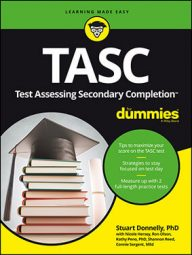##### TASC For DummiesIf you run across a system of equations problem on the TASC Math exam, two ways to solve it—if you decide to avoid graphing—are through substitution and elimination.

## Using the substitution method

To solve a system of two variables using the substitution method, follow these steps:
1. Solve one or both equations for one of the variables.
2. Substitute what one variable equals into the other equation.
3. Solve the resulting equation for that one variable.
4. Substitute the found value back into either original equation to solve for the second value.
You can also use this method to solve more than two variables, but it may require a bit more algebra because there will be more equations involved.

Try this example:

y = 2x + 5

3x – 4y = 10

Because the first equation is already solved for y, you substitute what y equals into the second equation.

Now that you have a value for x, you can substitute it into either of the original equations.

So the solution to the system is (–6, –7). You can check this solution by substituting both values into both original equations.

## Using the elimination method

To solve a system with two variables using the elimination method, follow these steps:
1. Rewrite both equations so their variables are in the same order.
2. One pair of variables must have the same coefficients but with opposite signs. For example, if one equation has 2x, then the other equation needs to have –2x. If there isn't an existing pair of coefficients that meet this requirement, you must multiply or divide one or both equations.
3. Add the equations together; the pair identified in Step 2 should cancel.
4. Solve the produced equation for the remaining variable.
5. Substitute the value obtained in Step 4 back into one of the original equations.
6. Solve for the other variable.
Try this example:

Notice how the y's fit the requirement in Step 2. Add the two equations together.

Now that you have a value for x, you can substitute it back into either of the original equations.

The solution to the system is (3, –1/3).

Stuart Donnelly, PhD, was awarded a PhD in mathematics from Oxford University. He has prepared students for the TASC test and GED Test for the past two decades.

Stuart Donnelly, PhD, earned his doctorate in mathe-matics from Oxford University at the age of 25. Since then, he has established successful tutoring services in both Hong Kong and the United States and is considered by leading educators to be one of the most experienced and qualified private tutors in the country.

Stuart Donnelly, PhD, was awarded a PhD in mathematics from Oxford University. He has prepared students for the TASC test and GED Test for the past two decades.

Stuart Donnelly, PhD, was awarded a PhD in mathematics from Oxford University. He has prepared students for the TASC test and GED Test for the past two decades.

Sandra Luna McCune, PhD, is professor emeritus and a former Regents professor at Stephen F. Austin State University. She's now a full-time author. Shannon Reed, MA, MFA, is a visiting lecturer at the University of Pittsburgh, where she teaches composition, creative writing, and business writing.

Stuart Donnelly, PhD, was awarded a PhD in mathematics from Oxford University. He has prepared students for the TASC test and GED Test for the past two decades.